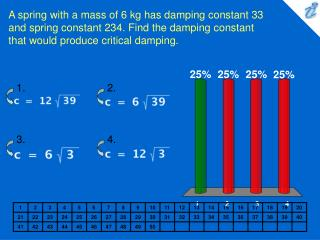DownloadDownload PresentationA spring with a mass of 6 kg has damping constant 33 and spring constant 234. Find the damping constant that would produ

A spring with a mass of 6 kg has damping constant 33 and spring constant 234. Find the damping constant that would produ

Télécharger la présentationA spring with a mass of 6 kg has damping constant 33 and spring constant 234. Find the damping constant that would produ

- - - - - - - - - - - - - - - - - - - - - - - - - - - E N D - - - - - - - - - - - - - - - - - - - - - - - - - - -
Presentation Transcript

1. 1. 2. 3. 4. A spring with a mass of 6 kg has damping constant 33 and spring constant 234. Find the damping constant that would produce critical damping. • {image} • {image} • {image} • {image}

2. 1. 2. 3. A spring has a mass of 1 kg and its damping constant is c = 10 . The spring starts from its equilibrium position with a velocity of 1 m/s . Graph the position function for the spring constant k = 10. • {applet} • {applet} • {applet}

3. 1. 2. 3. 4. A series circuit consists of a resistor {image} , an inductor with L = 2 H, a capacitor with C = 0.005 F, and a generator producing a voltage of E ( t ) = 12cos 10t . If the initial charge is Q = 0.001 C and the initial current is 0, find the charge Q ( t ) at time t. • {image} • {image} • {image} • {image}

4. 1. 2. 3. 4. A series circuit consists of a resistor {image} , an inductor with L = 1 H, a capacitor with C = 0.002 F, and a generator producing a voltage of E ( t ) = 12cos 10t. If the initial charge and current are both 0, find the charge Q ( t ) at time t. • {image} • {image} • {image} • {image}